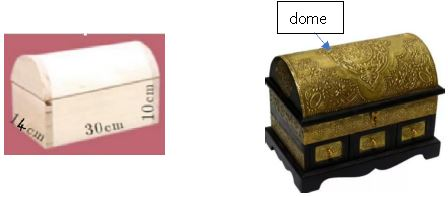# CBSE Class 9 Mathematics Surface Areas And Volumes Worksheet Set B

Read and download free pdf of CBSE Class 9 Mathematics Surface Areas And Volumes Worksheet Set B. Students and teachers of Class 9 Surface areas and Volumes can get free printable Worksheets for Class 9 Surface areas and Volumes in PDF format prepared as per the latest syllabus and examination pattern in your schools. Standard 9 students should practice questions and answers given here for Surface areas and Volumes in Grade 9 which will help them to improve your knowledge of all important chapters and its topics. Students should also download free pdf of Class 9 Surface areas and Volumes Worksheets prepared by school teachers as per the latest NCERT, CBSE, KVS books and syllabus issued this academic year and solve important problems with solutions on daily basis to get more score in school exams and tests

## Worksheet for Class 9 Surface areas and Volumes Chapter 13 Surface areas and Volumes

Class 9 Surface areas and Volumes students should refer to the following printable worksheet in Pdf for Chapter 13 Surface areas and Volumes in standard 9. This test paper with questions and answers for Grade 9 Surface areas and Volumes will be very useful for exams and help you to score good marks

### Class 9 Surface areas and Volumes Worksheet for Chapter 13 Surface areas and Volumes

CBSE Class 9 Mathematics Worksheet - Surface areas and Volumes - Practice worksheets for CBSE students. Prepared by teachers of the best CBSE schools in India.

Case study question- 1

An antique box and its dimensions excluding the stand is given below.Question. What is the volume of the jewellery box?
a) (𝑙×𝑏×ℎ) + 𝜋𝑟2
b) (𝑙×𝑏×ℎ) + 1/2 𝜋𝑟2
c) 2(𝑙𝑏×𝑏ℎ × 𝑙ℎ)+𝜋𝑟2
d) (𝑙×𝑏×ℎ) + 2𝜋𝑟ℎ

Question. How much brass will be needed to plate the curved surface of the dome as shown in figure?
a) 1320cm2
b) 220 cm2
c) 440 cm2
d) 660 cm2

Question. How many sheets of dimensions 14cm x 30cm x 2cm can be placed in the box?
a) 10
b) 5
c) 2
d) 15

Question. Considering the thickness of the box to be negligible, how much velvet cloth will be needed to cover the cuboidal inner area?
a) 1720 cm2
b) 880 cm2
c) 1300 cm2
d) 1580 cm2

Question. A circus tent is cylindrical up to a height of 3m and conical above it. If the diameter of the base is 105m and the slant height of the conical part is 53cm, find the total canvas required in making the tent.

Question. A bird-bath in a garden is in the shape of a cylinder with a hemi-spherical depression at one end. The height of the hollow cylinder is 1.45m and its radius is 30cm. find the TSA of the bird-bath.

Question. The sum of the radius of base and height of a solid right circular cylinder is 37 cm. If the total surface area of the solid cylinder is 1628 sq. cm, find the volume of the cylinder

Question. Two cubes each of side 4cm are joined end to end. Find the volume of the resulting solid.
128cm3

Question. Volume and surface area of a solid hemisphere are numerically equal. What is the diameter of hemisphere?
9units

Question. A metallic cylinder has radius 3cm and height 5cm. To reduce its weight, a conical hole is drilled in the cylinder. The conical hole is drilled in the cylinder. The conical hole has a radius of 3/2 cm. and its depth is 8/9cm. Calculate the ratio of the volume of metal left in the cylinder to the volume of metal taken out in the conical shape.

Question. A rectangular sheet of paper 30cm x 18cm can be transformed into the curved surface of a right circular cylinder in two ways either by rolling the paper along its length or by rolling it along its breadth. Find the ratio of the volume of the two cylinders thus formed.

Question. Find the volume of a right circular cylinder of base radius 7 cm and height 10 cm.
1540 cm3

Question. A right circular cylinder and a cone have equal bases and equal heights. If their curved surface areas are in the ratio 8:5, show that the ratio between the radius of their bases to their heights is 3:4

Question. The internal and external diameters of a hollow hemispherical vessel are 12cm and 16cm respectively. If the cost of painting 1 sq.cm of the surface area is Rs. 5, find the total cost of painting the vessel all over. (π = 3.14)
Rs. 3579.60

Question. The sum of the radius of the base and height of a solid right circular cylinder is 37cm. if the total surface area of the solid cylinder is 1628 sq.cm, find the volume of the cylinder. (π= 22/7)